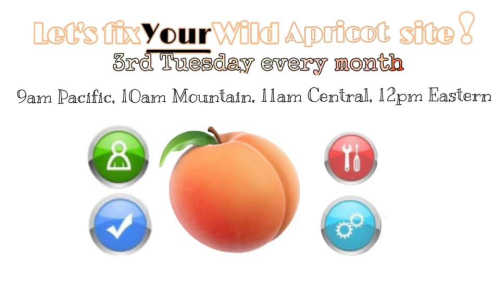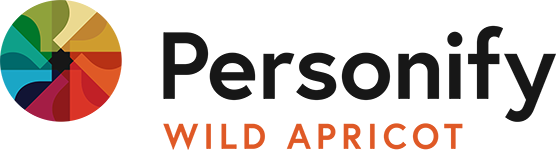/* styles */ Dear friend,
 /* styles */ It's February in Canada. It's damn cold. Brrrr. Are you hibernating? Maybe it's time to move? Dan Pink, my friend who is a famous author and speech writer, says exercise is important. But starting to exercise can be hard, as we all know. This 114-second video that offers a simple one-word trick to motivate yourself to exercise. In our product news, I am delighted to announce the Wild Apricot QuickBooks Manager for our Wild Apricot users who need better integration with QuickBooks Desktop and QuickBooks Online. Get it for only \$99.
 /* styles */ As always, feel free to reach out to me at alex@newpathconsulting.com to talk and connect. I look forward to hearing from you. Here is the February 2021 edition (#86) of the NewPath Consulting newsletter.
 table div table+table+table+table+table+table+table div table,table.module-5{width:35.28%;float:left;padding:0}table div table+table+table+table+table+table+table div table a{border:0 none;text-decoration:none}table div table+table+table+table+table+table+table div table img{width:100%!important;border:0 none;text-decoration:none}table div table+table+table+table+table+table+table div table td{width:100%;padding:0 20px 20px 0}/* styles */ Alex Sirota Director, NewPath Consulting (888) 781-2539

## Let's Fix Your Wild Apricot Website

 /* styles */ Do you have a problem you are facing or want to figure out something with Wild Apricot? Do you want a second opinion? Do you want to learn about what you can do with Wild Apricot from experts and other organizations? "Let's Fix Your Wild Apricot Site" is a free, live webconference sponsored by NewPath Consulting. It is hosted by NewPath Consulting every third Tuesday at 12pm ET. The next event is February 16, 2020.Let's Fix Your Wild Apricot Website
 table div table+table+table+table+table+table+table+table+table+table+table div table{width:100%;padding:0}table div table+table+table+table+table+table+table+table+table+table+table div table img{width:96.23%;padding:0;float:none}table div table+table+table+table+table+table+table+table+table+table+table div table td{width:100%;padding:0 1.88% 18px}/* styles */## Integromat TechTalk for Partners

Alex Sirota, Director of NewPath Consulting is hosting a monthly Integromat partner TechTalk #5.

All tech talks allow partners to meet each other and hear about exciting developments in the Integromat partner community.

All Integromat partners are welcome.

 table div table+table+table+table+table+table+table+table+table+table+table+table+table+table+table div table{width:100%;padding:0}table div table+table+table+table+table+table+table+table+table+table+table+table+table+table+table div table img{width:96.23%;padding:0;float:none}table div table+table+table+table+table+table+table+table+table+table+table+table+table+table+table div table td{width:100%;padding:0 1.88% 18px}/* styles */## Cool Resource of the Month - GSuite or Microsoft 365 FREE

 /* styles */ Did you know Google and Microsoft make their productivity software available for free? Gsuite for Not for Profits is available at no cost to eligible not for profits. That's \$72USD per seat per year in value! And if you need more than 30GB storage, the Business for Not for Profits is only \$4 USD / month. Microsoft 365 is also available for little to no cost for not for profits.
 table div table+table+table+table+table+table+table+table+table+table+table+table+table+table+table+table+table+table+table+table div table{width:100%;padding:0}table div table+table+table+table+table+table+table+table+table+table+table+table+table+table+table+table+table+table+table+table div table img{width:96.23%;padding:0;float:none}table div table+table+table+table+table+table+table+table+table+table+table+table+table+table+table+table+table+table+table+table div table td{width:100%;padding:0 1.88% 18px}/* styles */## NewPath Communiy

When we work from anywhere we require connection. The NewPath Community launched last month for all our customers and friends:

▪ To connect.
▪ To inspire.
▪ To learn.
▪ And to grow.
 ▪ To connect.
 ▪ To inspire.
 ▪ To learn.
 ▪ And to grow.
 table div table+table+table+table+table+table+table+table+table+table+table+table+table+table+table+table+table+table+table+table+table+table+table+table div table{width:100%;padding:0}table div table+table+table+table+table+table+table+table+table+table+table+table+table+table+table+table+table+table+table+table+table+table+table+table div table img{width:96.23%;padding:0;float:none}table div table+table+table+table+table+table+table+table+table+table+table+table+table+table+table+table+table+table+table+table+table+table+table+table div table td{width:100%;padding:0 1.88% 18px}/* styles */Get a free trial of Wild Apricot
 table.module-25{width:53.21%;padding:0}table div table+table+table+table+table+table+table+table+table+table+table+table+table+table+table+table+table+table+table+table+table+table+table+table+table+table+table div table{width:53.21%;float:none;margin-left:auto;margin-right:auto;padding:0}table div table+table+table+table+table+table+table+table+table+table+table+table+table+table+table+table+table+table+table+table+table+table+table+table+table+table+table div table a{border:0 none;text-decoration:none}table div table+table+table+table+table+table+table+table+table+table+table+table+table+table+table+table+table+table+table+table+table+table+table+table+table+table+table div table img{width:100%!important;border:0 none;text-decoration:none}table div table+table+table+table+table+table+table+table+table+table+table+table+table+table+table+table+table+table+table+table+table+table+table+table+table+table+table div table td{width:100%;padding:0}/* styles */
 table.module-27{width:48.11%;padding:0}table div table+table+table+table+table+table+table+table+table+table+table+table+table+table+table+table+table+table+table+table+table+table+table+table+table+table+table+table+table div table{width:48.11%;float:none;margin-left:auto;margin-right:auto;padding:0}table div table+table+table+table+table+table+table+table+table+table+table+table+table+table+table+table+table+table+table+table+table+table+table+table+table+table+table+table+table div table a{border:0 none;text-decoration:none}table div table+table+table+table+table+table+table+table+table+table+table+table+table+table+table+table+table+table+table+table+table+table+table+table+table+table+table+table+table div table img{width:100%!important;border:0 none;text-decoration:none}table div table+table+table+table+table+table+table+table+table+table+table+table+table+table+table+table+table+table+table+table+table+table+table+table+table+table+table+table+table div table td{width:100%;padding:0}/* styles */
 Like   Tweet   in